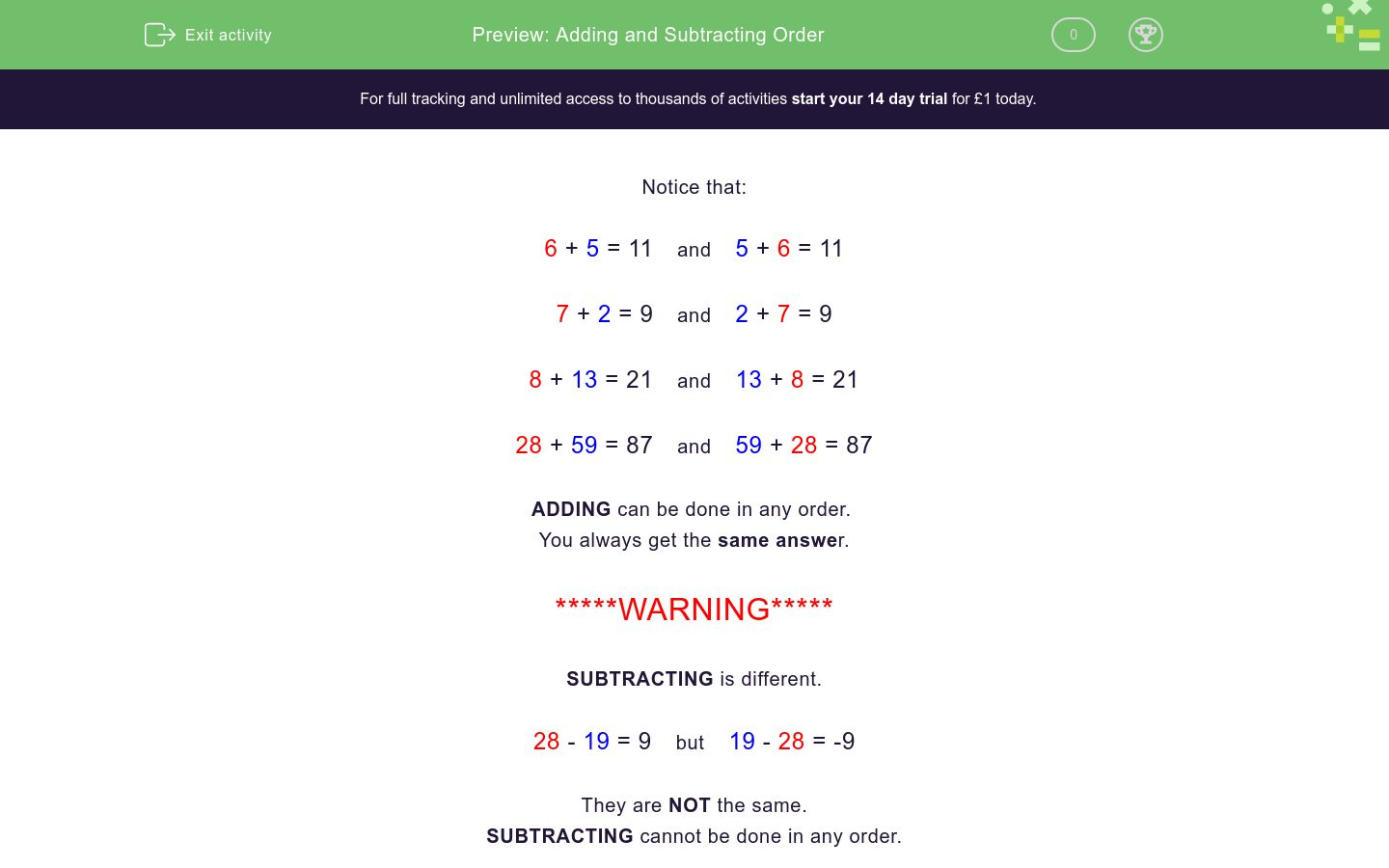### Comprehensive & curriculum aligned

In this worksheet, students must understand that addition can be done in any order but subtraction cannot.Key stage:  KS 1

Curriculum topic:   Number: Addition and Subtraction

Curriculum subtopic:   Understand Order for Addition/Subtraction

Difficulty level:### QUESTION 1 of 10

Notice that:

6 + 5 = 11    and    5 + 6 = 11

7 + 2 = 9    and    2 + 7 = 9

8 + 13 = 21    and    13 + 8 = 21

28 + 59 = 87    and    59 + 28 = 87

ADDING can be done in any order.

You always get the same answer.

*****WARNING*****

SUBTRACTING is different.

28 - 19 = 9    but    19 - 28 = -9

They are NOT the same.

SUBTRACTING cannot be done in any order.

## Column B

6 + 7
7 + 6
3 + 4
4 + 8
8 + 1
4 + 3
8 + 4
1 + 8

Is this true or false?

38 - 19  and  19 - 38  are the same.

true

false

15 + 45 is the same as...

45 + 15

55 + 45

54 + 14

37 - 12 is the same as...

37 + 12

12 + 37

12 - 37

none of these

51 + 28 = 79

What is  28 + 51?

23 - 4
7 + 16
16 + 7
14 + 28
28 + 14
no match
28 + 34
34 + 28

## Column B

23 + 48
48 + 23
23 + 31
31 + 23
31 - 28
no match
13 + 23
23 + 13

67 - 45  is the same as...

45 - 67

none of these

67 + 45

27 + 59 is the same as...

27 + 95

59 + 27

59 + 72

34 + 27 = 61

What is  27 + 34?

• Question 1

## Column B

6 + 7
7 + 6
3 + 4
4 + 3
8 + 1
1 + 8
8 + 4
4 + 8
EDDIE SAYS
Adding can be done in any order.
• Question 2

Is this true or false?

38 - 19  and  19 - 38  are the same.

false
EDDIE SAYS
Subtracting cannot be done in any order.
• Question 3

15 + 45 is the same as...

45 + 15
EDDIE SAYS
15 + 45 = 45 + 15
• Question 4

37 - 12 is the same as...

none of these
EDDIE SAYS
37 - 12 and 12 - 37 are not the same.
• Question 5

51 + 28 = 79

What is  28 + 51?

79
EDDIE SAYS
It is the same.
• Question 6

## Column B

23 - 4
no match
16 + 7
7 + 16
28 + 14
14 + 28
28 + 34
34 + 28
EDDIE SAYS
Adding can be done in any order.
• Question 7

## Column B

23 + 48
48 + 23
23 + 31
31 + 23
31 - 28
no match
13 + 23
23 + 13
EDDIE SAYS
Adding can be done in any order.
Subtracting cannot.
• Question 8

67 - 45  is the same as...

none of these
EDDIE SAYS
Subtracting cannot be done in reverse order.
• Question 9

27 + 59 is the same as...

59 + 27
EDDIE SAYS
27 + 59 = 59 + 27
• Question 10

34 + 27 = 61

What is  27 + 34?

61
EDDIE SAYS
It is the same.
---- OR ----